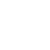# Squares of a Sorted Array LeetCode O(N)

In this problem, given a sorted array in decreasing order and we should return the squares of each number and in the same ascending order, and the one thing we need to take care of is that the negative numbers, which when squared disturb the ascending ordered array.

## Problem Statement

Given an array of integers A sorted in non-decreasing order, return an array of the squares of each number, also in sorted non-decreasing order.

## Examples

``````Input: [-5,-1,0,3,10]
Output: [0,1,9,25,100]

Input: [-7,-2,2,3,11]
Output: [4,4,9,49,121]``````

## Solution Approach

We can solve this in many ways like just get the square of all elements and then append them to a new array and then sort them out but, let’s complete this in a single iteration

We need to sort them in ascending order, but they are already in decreasing/ascending order. However, there are chances for the negative elements at the beginning of the sorted array to be greater than the last element when squared, for example

``````[-9,0,1,7 ]
[81,0,1,49] [after squaring]
[0,1,49,81]``````

So we start filling the array from the end and before that, we compare array elements both from the front and the back preserving the order. Here we actually use extra space but, we’re using a single iteration i.e saving a lot of time on the bright side.

• Initialize a new array of size N
• Compare the elements with abs ()- absolute value
• Put the largest element at the end of the array and square it
• Repeat for all

## Code – Squares of a Sorted Array LeetCode C++

``````class Solution {
public:
vector<int> sortedSquares(vector<int>& A)
{
vector<int> res(A.size());
int l = 0, r = A.size() - 1;
for (int k = A.size() - 1; k >= 0; k--) {
if (abs(A[r]) > abs(A[l]))
res[k] = A[r] * A[r--];
else
res[k] = A[l] * A[l++];
}
return res;
}
};``````

## Conclusion

This post is part of my #30DaysChallenge to write a blog post every day on what I learn. When I first tried to solve this problem, I did the long way but then I felt this method saves a lot of time, however if you find much optimized or improved solution feel free to share it here, cheers~ Abhiram Reddy

Link to Squares of a Sorted Array LeetCode

## 2 thoughts on “Squares of a Sorted Array LeetCode O(N)”

1.Intelligent way Abhi, Keep continuing the same.

1.Thank You!